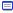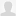# 雲雀は高く空を舞い「ひよこは高く空を舞い」について

## 2007-11-12

### ■```// バッチ学習による自己組織化マップ
// data:入力データ[dimension_number * sample_number]
// dimension_number:入力データの次元数
// sample_number:入力データの個数
// iterate:学習回数
// node:ノード[dimension_number * grid_width * grid_height]
// grid_width:格子の横幅
// grid_height:格子の高さ
// map:入力データがどのノードに属するか[2 * sample_number]
void BatchSelfOrganizingMap(double *data, int dimension_number, int sample_number, int iterate, double *node, int grid_width, int grid_height, int *map)
{
int index = 0;
int *label = new int [sample_number];
int *node_size = new int [grid_width * grid_height];
double *temp_node = new double [dimension_number * grid_width * grid_height];
double tolerance = 1e-8;
double distance;
double sum_distance;
double min_distance;
double old_error = 0.0;
double new_error = 0.0;

// 初期値を設定する．
srand(time(NULL));
for (int y = 0; y < grid_height; y++) {
for (int x = 0; x < grid_width; x++) {
index = (int)(((double)rand() / RAND_MAX) * (sample_number - 1));
for (int d = 0;  d < dimension_number; d++) {
node[(d * grid_height + y) * grid_width + x] = data[d * sample_number + index];
}
}
}

// 学習を開始する．
for (int t = 0; t < iterate; t++) {
// 初期化する．
old_error = new_error;
new_error = 0.0;

// 各データがどのノードに属するかを求める．
for (int n = 0; n < sample_number; n++) {
// 距離が近いノードにデータを割り当てる．
min_distance = DOUBLE_MAX;
for (int y = 0; y < grid_height; y++) {
for (int x = 0; x < grid_width; x++) {
distance = 0.0;
for (int d = 0; d < dimension_number; d++) {
distance += (data[d * sample_number + n] - node[(d * grid_height + y) * grid_width + x]) * (data[d * sample_number + n] - node[(d * grid_height + y) * grid_width + x]);
}
if (distance < min_distance) {
min_distance = distance;
map[0 * sample_number + n] = x;
map[1 * sample_number + n] = y;
}
}
}
}

// 近傍ノードに属するデータでノードを更新する．
memset(node_size, 0, grid_width * grid_height * sizeof(int));
memset(temp_node, 0, dimension_number * grid_width * grid_height * sizeof(double));

for (int y = 0; y < grid_height; y++) {
for (int x = 0; x < grid_width; x++) {
for (int n = 0; n < sample_number; n++) {
if ((map[0 * sample_number + n] == x) && (map[1 * sample_number + n] == y)) {
for (int d = 0; d < dimension_number; d++) {
temp_node[(d * grid_height + y) * grid_width + x] += data[d * sample_number + n];
}
node_size[y * grid_width + x]++;
}
if ((map[0 * sample_number + n] == x) && (map[1 * sample_number + n] == y - 1)) {
for (int d = 0; d < dimension_number; d++) {
temp_node[(d * grid_height + y) * grid_width + x] += data[d * sample_number + n];
}
node_size[y * grid_width + x]++;
}
if ((map[0 * sample_number + n] == x) && (map[1 * sample_number + n] == y + 1)) {
for (int d = 0; d < dimension_number; d++) {
temp_node[(d * grid_height + y) * grid_width + x] += data[d * sample_number + n];
}
node_size[y * grid_width + x]++;
}
if ((map[0 * sample_number + n] == x - 1) && (map[1 * sample_number + n] == y)) {
for (int d = 0; d < dimension_number; d++) {
temp_node[(d * grid_height + y) * grid_width + x] += data[d * sample_number + n];
}
node_size[y * grid_width + x]++;
}
if ((map[0 * sample_number + n] == x + 1) && (map[1 * sample_number + n] == y)) {
for (int d = 0; d < dimension_number; d++) {
temp_node[(d * grid_height + y) * grid_width + x] += data[d * sample_number + n];
}
node_size[y * grid_width + x]++;
}
}
}
}

for (int y = 0; y < grid_height; y++) {
for (int x = 0; x < grid_width; x++) {
if (node_size[y * grid_width + x] > 0) {
for (int d = 0; d < dimension_number; d++) {
temp_node[(d * grid_height + y) * grid_width + x] = temp_node[(d * grid_height + y) * grid_width + x] / (double)node_size[y * grid_width + x];
}
} else {
for (int d = 0; d < dimension_number; d++) {
temp_node[(d * grid_height + y) * grid_width + x] = node[(d * grid_height + y) * grid_width + x];
}
}
}
}

// ノードに変化がなければ終了する．
new_error = 0.0;
for (int y = 0; y < grid_height; y++) {
for (int x = 0; x < grid_width; x++) {
distance = 0.0;
for (int d = 0; d < dimension_number; d++) {
distance += (temp_node[(d * grid_height + y) * grid_width + x] - node[(d * grid_height + y) * grid_width + x]) * (temp_node[(d * grid_height + y) * grid_width + x] - node[(d * grid_height + y) * grid_width + x]);
}
new_error += distance;
}
}

if (fabs(new_error - old_error) < tolerance) {
break;
}

// 次の学習へ．
for (int i = 0; i < dimension_number * grid_width * grid_height; i++) {
node[i] = temp_node[i];
}
}

// おしまい
delete [] label;
delete [] temp_node;
delete [] node_size;
}
```rtfgmc2011/03/04 23:12CRtc0X <a href="http://qwfsrmpvjndp.com/">qwfsrmpvjndp</a>, [url=http://npykvigwkeep.com/]npykvigwkeep[/url], [link=http://zqfxvgxsdhpo.com/]zqfxvgxsdhpo[/link], http://mvihzcpqhmgv.com/Dilyaram2013/05/05 19:23Essays like this are so important to broadening people's hrozinos.wvqjwdhlks2013/05/08 01:33hIPWmX , [url=http://varoqzwwtqvx.com/]varoqzwwtqvx[/url], [link=http://dzbrtkauwmji.com/]dzbrtkauwmji[/link], http://tgqlwpvfupyf.com/isxjggslb2013/05/08 07:5364R2FZ <a href="http://lapnjfpjdlki.com/">lapnjfpjdlki</a>tluuxfgz2013/05/12 13:13Gn7utQ , [url=http://uedzdlmejrvl.com/]uedzdlmejrvl[/url], [link=http://mkbjfnjyvdjc.com/]mkbjfnjyvdjc[/link], http://waslkpxlstuq.com/

トラックバック - http://chick.g.hatena.ne.jp/allegro/20071112テクノラティプロフィール